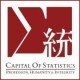# 用R测量灯泡的体积

## 阿普顿其人## 亲历者版本的测量灯泡故事

“I was once with Mr Upton calculating some tables he had put me on, when Mr Edison appeared with a glass bulb having a pear-shaped appearance in his hand. It was the kind we were going to use for our lamp experiments; and Mr Edison asked Mr Upton to please calculate its cubical content in centimetres. Now Mr Upton was a very able mathematician, who after he finished his studies at Princeton went to Germany and got his final gloss under the great master Helmholtz. Whatever he did and worked on was executed in a purely mathematical manner and any Wrangler at Cambridge would have been delighted to see him juggle with integral and differential equations with a dexterity that was surprising. He drew the shape of the bulb exactly on paper, and got the equation of its lines with which he was going to calculate its contents, when Mr Edison again appeared and asked him what it was. He showed Mr Edison the work he had already done on the subject and told him he would very soon finish calculating it. “Why,” said Edison, “I would simply take that bulb and fill it with mercury and weigh it; and from the weight of the mercury and its specific gravity, I’ll get it in five minutes, and use a lot less mental energy than is necessary in such a fatiguing operation.”

“我有一次正和阿普顿先生计算一些他拿过来的数据表。这时，爱迪生先生出现了。他手里拿着一个梨子形状的灯泡，这正是我们准备进行电灯实验的那种型号。爱迪生先生请求阿普顿先生计算一下灯泡的容积是多少立方厘米。阿普顿先生现在已经是一名非常出色的数学家了，他曾在普林斯顿大学完成学业，又去过德国，在大牛 Helmholtz 那里更近层楼，数学方面已经很厉害了。他在以纯数学的方式解决问题的时候，灵活熟练地在微积分方程间闪辗腾挪，哪怕是任何一名剑桥大学毕业的牛仔都会羡慕惊艳。阿普顿先生首先在纸上准确第勾勒出灯泡的形状。然后他用方程拟合好了轮廓曲线，正要根据这些结果计算体积的时候,爱迪生先生就回来询问结果了。阿普顿先生展示了已经做的，并告诉他将很快完成计算。’为什么？’爱迪生说，‘我会简单地在灯泡中注入汞并称重，根据汞的重量及其密度，5分钟就会得到灯泡的体积。比起这项累人的计算，要省下不少的脑力。’”

## 阿普顿的计算方法

1. 勾勒灯泡轮廓曲线；
2. 对曲线进行方程拟合；
3. 根据曲线方程，进行积分，求体积。

## R语言计算灯泡体积

### 1. 勾勒灯泡轮廓曲线

realx=function(x){(x-68)*140/(442-68)}
realy=function(y){(y-283)*140/(442-68)}


library(png)
library(ggplot2)

myaddr$cols=apply(myaddr,1,function(x){ rgb(mypic[x,x,1], mypic[x,x,2], mypic[x,x,3])}) myaddr$x=realx(myaddr$x) myaddr$y=realy(myaddr$y) pic=ggplot(myaddr) + geom_raster(aes(x,y,fill=cols)) + geom_hline(aes(yintercept=tk), colour="grey",alpha=0.5, data=data.frame(tk=c(-100,-50,0,50))) + geom_vline(aes(xintercept=tk), colour="grey",alpha=0.5, data=data.frame(tk=c(0,50,100,150))) + geom_hline(yintercept=0,colour="white") + scale_fill_identity() + coord_fixed() + scale_x_continuous(expand=c(0,0)) + scale_y_continuous(expand=c(0,0)) + labs(x="Length (mm)", y="Width (mm)") print(pic)### 2. 对曲线进行方程拟合 我们首先用gimp对原始照片中灯泡的边缘选取一些点采样坐标，并转化为真实坐标。 mypoints=data.frame(y=c(283,304,320,337,354,374,388,385,368,344,341,339,329), x=c( 68, 70, 74, 82, 95,120,168,202,240,289,311,334,352)) mypoints$x=realx(mypoints$x) mypoints$y=realy(mypoints$y) mypoints  ## y x ## 1 0.000 0.0000 ## 2 7.861 0.7487 ## 3 13.850 2.2460 ## 4 20.214 5.2406 ## 5 26.578 10.1070 ## 6 34.064 19.4652 ## 7 39.305 37.4332 ## 8 38.182 50.1604 ## 9 31.818 64.3850 ## 10 22.834 82.7273 ## 11 21.711 90.9626 ## 12 20.963 99.5722 ## 13 17.219 106.3102  对采样点进行三次样条插值，对轮廓进行曲线拟合： library(splines) myfx=interpSpline(mypoints$x,mypoints$y)  整个第二部分的工作，曲线拟合已经做完了，整个第二部分就只需要一行语句负责计算！ 方程曲线保存在自变量myfx中。 曲线采样分段拟合，每段采样三次多项式表示： y = A + Bx + Cx2 + Dx3 , x[i] ≤ x ＜ x[i+1] 后续的编程中，不必具体知道这些系数究竟是多少。非要查看的话，在自定义变量myfx中，可以查看，这些方程的系数分别是： tmpdf=as.data.frame(myfx$coefficients); tmpdf$V5=(myfx$knots);
names(tmpdf)<-c("A","B","C","D","x[i]"); kable(tmpdf,"html");

A B C D x[i]
0.000 11.5973 0.0000 -1.9577 0.0000
7.861 8.3054 -4.3970 1.0162 0.7487
13.850 1.9730 0.1678 -0.0391 2.2460
20.214 1.9265 -0.1834 0.0116 5.2406
26.578 0.9625 -0.0147 -0.0003 10.1070
34.064 0.6130 -0.0226 0.0003 19.4652
39.305 0.0554 -0.0084 -0.0002 37.4332
38.182 -0.2684 -0.0170 0.0003 50.1604
31.818 -0.5630 -0.0037 0.0004 64.3850
22.834 -0.2761 0.0193 -0.0003 82.7273
21.711 -0.0160 0.0123 -0.0024 90.9626
20.963 -0.3344 -0.0492 0.0024 99.5722
17.219 -0.6661 0.0000 0.0000 106.3102

mysmooth=as.data.frame(predict(myfx, x=seq(0, max(mypoints$x), length.out=100))) pic=pic + geom_line(aes(x,y), size=1.3, colour="yellow", data=mysmooth) + geom_point(aes(x,y), shape=13,size=5,colour="white",data=mypoints) + annotate("text",x=20,y=-60,label="V==integral(pi*f(x)^2*dx,a,b)", size=8, colour="white", parse=TRUE, hjust=0) + annotate("text",x=20,y=-85,label="f(x)=Spline of Points", size=6, colour="white", hjust=0) print(pic)可以看到，曲线完美拟合灯泡的轮廓。 ### 3. 根据曲线方程，进行积分，求体积。 V=integrate(function(x){pi*(predict(myfx,x)$y)^2}, 0, max(mypoints\$x))
print(V)

## 311680 with absolute error < 34


## 结尾

100年后的今天，我们有了R语言，不再需要懂复杂的技巧和高深的理论，就可以轻松完成这些工作。不过,却再也没有微积分闪耀在稿纸上的灵动，再也没有剑桥牛仔投向阿普顿的热切眼神了。我们只能在文献的字里行间，隐约感觉到折射出来的人性光辉，在心底投以满满的敬意。

## 参考文献

1. 维基百科, Francis Robbins Upton, http://en.wikipedia.org/wiki/Francis_Robbins_Upton
2. J J O’Connor and E F Robertson, Francis Robbins Upton, http://www-history.mcs.st-andrews.ac.uk/Biographies/Upton.html## 《用R测量灯泡的体积》有5个想法

1.ttang说道：

疯狂的世界成就疯狂的理科生，疯狂的理科生让世界更疯狂…

1.子辰说道：

楼主像素换算的时候错了，分母应该是（图像长度-灯泡顶点到图像顶边的距离-灯泡底端到图像底边的距离），楼主少减了灯泡底端到图像底边的距离，因此灯泡体积被低估了。

1.doctorjxd说道：

子辰兄误解了，442并非是图像长度，已经做了扣除。A19.png原始图的大小是500×500像素。看看ggplot2绘出图的横坐标，就知道灯泡的长度是在140mm，换算是正确的。

2.itellin说道：

R的应用又扩展了一个领域，什么A,B罩杯过时了，现在可以直接测量重量了，同时还可以把轮廓画出来，穿着暴露的要小心了，大数据时代，无隐私了。

3.wangbinzjcc说道：

太牛了！佩服。